# KSEEB Solutions for Class 9 Science Chapter 8 Motion

Students can Download KSEEB Solutions for Class 9 Science Chapter 8 Motion, Karnataka State Board Solutions help you to revise complete Syllabus and score more marks in your examinations.

## Karnataka Board Class 9 Science Chapter 8 Motion

### KSEEB Solutions for Class 9 Science Chapter 8 Intext Questions

Our Displacement Calculator helps determine your engine’s size by its bore and stroke. This is a very useful tool for building high-performance racing engines.

Question 1.
An object has moved through a distance can it have zero displacement? If yes, support your answer with an example.In the figure distance of an object moved from 0 to A is 60 km and distance travelled is 60 km and displacement is 60 km. From 0 to A and back to B, distance travelled is 60 km + 25 km = 85 km. But displacement (35 km) is not equal to distance travelled (85 km). If we observe like this, displacement of a body is zero, but distance travelled is not zero, starting from point ‘0’ and returning back to ‘O’ final position is mixing with initial point. Hence displacement is zero.

Displacement Calculator is a free online tool that displays the displacement of a given object.

Question 2.
A farmer moves along the boundary of a square field of side 10 m in 40s. What will be the magnitude of displacement of the farmer at the end of 2 minutes 20 seconds from his initial position?
A farmer moves along the boundary of a square field of side 10 m in 40s
2 minutes 20 seconds means 140 seconds.
Distance travelled by farmer
= $$\frac{40}{40}$$ × 140 m
= 140 m.
∴ Farmer moves for 2 minutes 20 seconds. It means $$\frac{140}{40}$$ = 3.5 rounds
From the original point, he moves for 2 minutes 20 seconds.
Case i)
Original point means any point in the corner of the field. In this case he moves for 2 min 20 seconds from the diagonal of the field.
Displacement is equal to diagonal of the field displacement
= $$\sqrt{10^{2}+10^{2}}$$
= 14.1 m.
Case ii)
Original point means any point in the middle of the side of the field.
∴ Displacement is equal to any side of the field = 10 m.
It means displacement is between any original point ie in between 14.1 m and 10 m.

Question 3.
Which of the following is true for displacement?
a) It cannot be zero.
b) Its magnitude is greater than the distance travelled by the object.
b) Its magnitude is greater than the distance travelled by the object.

Question 4.
Distinguish between speed and velocity

 Speed Velocity 1. Scalar quantity Vector quantity 2. Distance travelled in a given time. Distance travelled along with a path with the given time. 3. It is always is + ve. It is + ve or – ve

Average velocity calculator is really nice tool to use and it will help you solve all your problem related to average velocity.

Question 5.
Under what condition (s) is the magnitude of the average velocity of an object equal to its average speed?
If the speed of the object changing uniformly, the magnitude of the average velocity of an object equal to its average speed.

Question 6.
What does the odometer of an automobile measure?
Odometer measures the speed of the vehicle.

Question 7.
What does the path of an object look like when it is in uniform motion?
When the object is in uniform motion, it covers equal distance at equal intervals of time.

Question 8.
During an experiment, a signal from a spaceship reached the ground station in five minutes. What was the distance of the spaceship from the ground station? The signal travels at the speed of light, which is 3 × 10 ms-1.
Time taken by the signal to reach the ground station from the spaceship = 5 min = 5 × 60 = 300 seconds
Speed of the signal = 3 × 108 m/s.
Speed = $$\frac{\text { Total distance travelled }}{\text { Total time taken }}$$
∴ Distance travelled = speed × time taken
= 3 × 108 × 300
= 9 × 1010m
∴ Distance of change of velocity = 9 × 1010 m

A versatile acceleration calculator with which you can calculate the acceleration given initial and final speed and acceleration time.

Question 9.
When will you say a body is in

1. Uniform acceleration?
2. non-uniform acceleration?

1. If the object covers equal distances in equal intervals of time, it is added to be in uniform motion.
2. If the object covers unequal distances in equal intervals of time, it is said to be in non-uniform motion.

Question 10.
A bus decreases its speed from 80 km h-1 to 60 km h-1 in 5s. Find the acceleration of the bus.
Initial velocity of the bus u = 80 kmh-1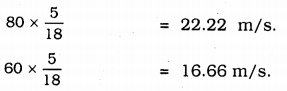Final velocity, V = 60 km/h
time taken to decrease the velocity of bus, t = 5 seconds
acceleration, a = 5 seconds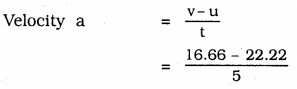= -1.112 ms-2.

Question 11.
A train starting from a railway station and moving with uniform acceleration attains a speed 40 km h-1 in 10 minutes. Find its acceleration.
Initial speed of the train u = 0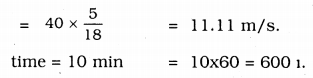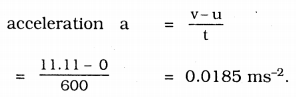∴ acceleration of a train = 0.0185 ms-2.

Question 12.
What is the nature of the distance-time graphs for uniform and non-uniform motion of an object?
(i) The nature of the distance-time graphs for the uniform motion of an object is a straight line.(ii) The nature of tire distance time graph for non-uniform motion is curved line.Distance-time graph for a car moving with non-uniform speed

Question 13.
What can you say about the motion of an object whose distance-time graph is straight line parallel to the time axis?
This graph indicates the object is at rest.

Question 14.
What can you say about the motion of an object if its speed-time graph is a straight line parallel to the time axis?
This graph indicates the object is in uniform motion.

Question 15.
What is the quantity which is measured by the area occupied below the velocity-time graph?
The quantity which is measured by the area occupied below the velocity-time graph is length = l

Question 16.
A bus starting from rest moves with a uniform acceleration of 0.1 ms-2 for 2 minutes. Find
(a) the speed acquired,
(b) the distance travelled.
(a) Initial velocity of the bus u = 0 (Bus is at rest).
acceleration, a = 0.1 ms-2
time t = 2 minutes = 120 seconds
Let the speed of bus be ‘V’∴ v = 12m/s
(b) As per third law of motion:
v2 – u2 = 2as
(12)2 – (0)2 = 2(0. 1)s
∴ s = 720 m.
the speed of acquired by bus = 12 m/s.
the distance travelled = 720 m.

Question 17.
A train is travelling at a speed of 90 kmh-1 Brakes are applied so as to produce a uniform acceleration of -0.5 ms-2. Find how far the train will go before it is brought to rest.
Let the initial speed of the train be u = 90 Km/h
= 25 m/s.
Final speed of the train, v = 0 (train comes to rest)
acceleration a = 0.5 ms-2
As per 3rd law of motion
v2 = u2 + 2as
(0)2 = (25)2 + 2(0.5)s
s = train travelled distanceTrain will travel 625 km before it is brought to rest.

Question 18.
A trolley, while going down an inclined plane has an acceleration of 2cms-2 what will be its velocity 3s after the start?
Initial velocity of a trolley, u = 0 (at rest)
acceleration, a = 2 cms-2 = 0.02 m/s2 time t = 3S
As per 1 st law of motion
v = u + at
Here V means velocity of a trolley after 3 s
V = 0 + 0.02 × 3
= 0.06 m/s.
∴ = 0.06 m/s.

Question 19.
A racing car has a uniform acceleration of 4ms-2, what distance will it cover in 10s after start?
Initial velocity of a racing car, u = 0 (at rest)
acceleration, a = 4 m/s2
time t = 10 s
As per second law of motionHere S means distance travelled by car after 10S∴ Distance covered by car after 10 S = 200 m.

Question 20.
A stone is thrown in a vertically upward direction with a velocity of 5ms-1 If the acceleration of the stone during its motion is 10ms-2 in the downward direction, what will he the height attain by the stone and how much time will it take to reach there?
The initial velocity of a stone, u = 5 ms-1.
Final velocity of a stone v = 0 (at rest)
If the acceleration of the stone during its motion is = -10ms-2.
Let the maximum height be ‘s’
v = u + at
0 = 5 + (-10)t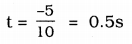time taken by stone = 0.5s
As per 3rd Law of motion
v2 = u2 + 2as
(0)2 = (5)2 + 2(-10)s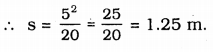The height attained by the store = 1.25 m.

### KSEEB Solutions for Class 9 Science Chapter 8 Textbook Exercises

Question 1.
An athlete completes one round of a circular track of diameter 200 m. in the 40s. What will be the distance covered and the displace¬ment at the end of 2 minutes 20s?
Diameter of circular track, d = 200 m.
r = $$\frac{\mathrm{d}}{2}$$ = 100m
Circumference = 2πr = 2p(100) = 200πcm.
in 40s, athlete covers distance 200πm
in 1s, distance covered by athlete = $$\frac{200 \pi}{40}$$ m
Athlete runs for 2 minutes 20s means 140s
∴ Displacement of all athlete is equal to the diameter of circle
= $$\frac{200 \times 22}{40 \times 7}$$ × 140
= 2200 m
∴ Displacement = 200 m.

Question 2.
Joseph jogs from one end A to the other end B of a straight 300 M road in 2 minutes 30 seconds and then turns around and jogs 100 M back to point C in another 1 minute. What are Joseph’s average speeds and velocities in jogging (a) from A to B and (b) from A to C?
a) from A to B distance covered by Joseph = 300 M.
Time taken to cover this distance
= 2 min 30 sec. = 150 seconds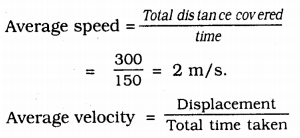Displacement = Nearest distance between A and B = 300 m.
Time taken = 150s
Average velocity = ygy = 2 m/s.

b) From A to C :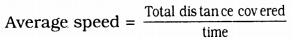Total distance covered = Distance between A to B + distance between B to C
= 300 + 100 = 400 m.
Total time taken to cover from A to B + Time taken to cover from B to C
= 150 + 60= 210s.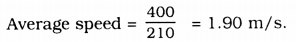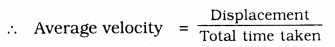Displacement from A to C
= AC + AB – BC
= 300 – 100
= 200m
Total time taken = Time taken to travel from A to B + Time taken to travel from B to C
= 210 s
∴ Average velocity = $$\frac{200}{210}$$ =0.952 ms-1 .

Question 3.
Abdul, while during to school, computes the average speed for his trip to be 20Kmh-1 on his return trip along the some route, there is less traffic and the average speed is 30 Kmh-1. What is the average speed for Abdul’s trip?
i) Average speed of Abdul’s trip while driving to school = 20 Kmh-1
Average speed = $$\frac{\text { Total distance }}{\text { Total time taken }}$$
Total distance = Distance travelled to reach school = t1
∴ 20 = $$\frac{\mathrm{d}}{\mathrm{t}_{1}}$$
t1 = $$\frac{d}{20}$$ …………… (i)
While returning from school
Total distance = Distance travelled while returning from school = d Now total time taken = t2.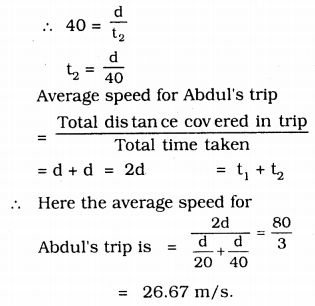Question 4.
A motorboat starting from rest on a lake accelerates in a straight line at a constant rate of 3.0 ms-2 for 8.0 s. How far does the boat travel during this time?
Initial velocity of motor boat, u =0
(at rest) ,
Accieratlon of a motor boat a = 3m/s2
Time taken, t = 85
According to second equation of motion, s
S = 0 +$$\frac{1}{2}$$ × (8)2 = 96m.
∴ Motorboat travels = 96m.

Question 5.
A driver of a car travelling at 52kmh-1 applies the brakes and accelerates uniformly in the opposite direction. The car stops in 5s. Another driver going at 3kmh-1 in another car applies his brakes slowly and stop In 10s. on the same graph paper. Plot the speed versus time graphs for the two cars, which of the two cars travelled farther after the brakes were applied?
initial velocity of A car u1 = 52 kmh-1
= 14.4 m/s.
Time taken to stop car t1 = 5 s.
After 5s car comes to rest Initial velocity of B car, u2 = 3Kmh-1
= 0.833 m/s. = 0.83 m/s.
Time taken to stop car t2 = 10s
After 10s car comes to rest.
Graph: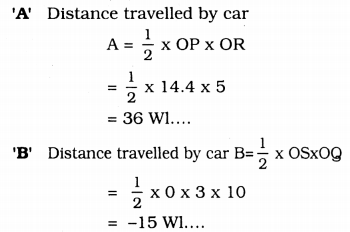Area of ∆OPR = Area of ∆OSQ
When compared car A to car B, A car travelled father. After brake it travels 52 Kmh-1

Question 6.
Fig 8.11 shows the distance-time graph of three objects A, B and C. Study the graph and answer the following questions.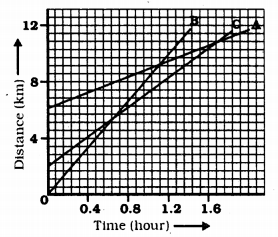a) Which of the three is travelled the fastest?
b) Are all three ever at the same point on the road?
c) How far has C travelled when B passes A?
d) How far has B travelled by the time passes C?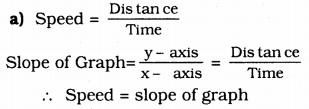Slope of B object is greater than slopes A and C. This is travelling fastest.

b) All three objects never meet at a point, hence these are not at the same point.

c) There are 7 boxes in X-axis = 4 Km
1 box = $$\frac{4}{7}$$ Km
In the beginning, C object is 4 boxes away from 0
= $$\frac{16}{7}$$ km
Distance to C from origin = Distance of C when it moves from B to A =8 Km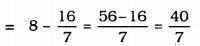∴ Distance travelled by C when B passes A = 5.714 km.

d) Distance B travelled by the time it passes C = 9 boxes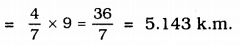∴ B has travelled 5.143 k.m. by the time when it passes C.

Question 7.
A ball is gently dropped from a height of 20 m. If its velocity increases uniformly at the rate of 10ms-1 with what velocity will it strike the ground? After what time will it strike the ground?
Distance ball covers, S = 20 m
acceleration, a = 10Kmh-1 = 10 m/s
initial velocity, u = 0 (at rest)
When ball strikes, its final velocity,
According to 3rd law of motion
v2 = u2 + 2as
v2 = 0 + 2(10)(20)
v = 20 m/s.
According to 1 st Law of motion v = u + at
Time taken by ball to strike the ground, t,
20 = 0 + 10 (t)
∴ t = 2s
∴ 2s is required to ball for striking the ground and velocity is 20 m/s

Question 8.
The speed-time graph for a car is shown in figure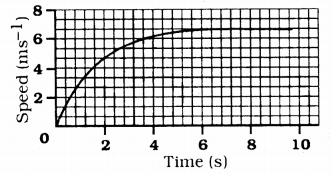a) Find how far does the car travel in the first 4 seconds. Shade the area on the graph that represents the distance travelled by car during the period.
b) Which part of the graph represents uniform motion of the car?
a) Shade lightly in the graph about distance travelled by car in 4s.
b) Shade with red colour about 6 cm to 10 cm. It represents the uniform motion of the car.

Question 9.
State which of the following situations are possible and give an example for each of these;
a) an object with a constant acceleration but with zero velocity.
b) an object moving in a certain direction with an acceleration in the perpendicular direction.
a) This is possible
Eg: when a ball is thrown to a height its velocity is zero.
(For a ball G = 9.8 m/s2)

b) This is possible
Eg: when a car moves in a curved line, its acceleration is perpendicular to the given direction)

Question 10.
An artificial satellite is moving in a circular orbit of radius 42250 km. Calculate its speed if it takes 24 hours to revolve around the earth.
Satellite is moving in circular orbit radium of satellite r = 42250 km
time t = 24 Hrs = 3600 × 24 S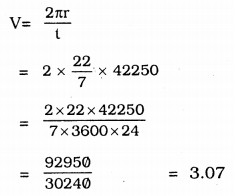∴ Speed of the satellite when it moves in circular orbit = 3.07 kms-1.

Mile calculator. Enter your route details and price per mile, and total up your distance and expenses

### KSEEB Solutions for Class 9 Science Chapter 8 Additional Questions

Fill in the blanks with suitable words.

Question 1.
The two physical quantities required to know final position of an object are _________ and _________
Distance travelled, displacement.

Question 2.
S.I. unit of speed is ________
m/s

Question 3.
Distance travelled by the object in unit time is ________
Speed.

Question 4.
If acceleration is in the direction of velocity it is _________ acceleration.
positive.

Question 5.
When an object moves in a circular path with uniform speed, its motion is called ________
uniform circular motion.

Question 1.
Give an express10n for centripetal force?
$$f=\frac{m v^{2}}{r}$$

Question 2.
What is uniform circular mot10n?